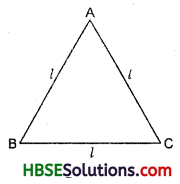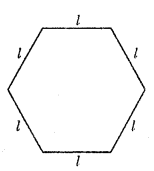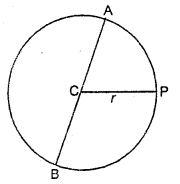# HBSE 6th Class Maths Solutions Chapter 11 Algebra Ex 11.2

Haryana State Board HBSE 6th Class Maths Solutions Chapter 11 Algebra Ex 11.2 Textbook Exercise Questions and Answers.

## Haryana Board 6th Class Maths Solutions Chapter 11 Algebra Exercise 11.2

Question 1.
The side of an equilateral triangle is shown by ‘l’. Express the perimeter of the equilateral triangle using T.Solution:
Perimeter of the equilateral ΔABC
= AB + BC + AC
= I + l + l
= 3l.

Question 2.
The side of a regular hexagon (Fig.) is denoted by l. Express the peri¬meter of the hexagon using l.
(Hint : A regular hexagon has all its six sides equal in length.)Solution:
Perimeter of a regular hexagon = l + l + l + l + l + l
= 6l.Question 3.
A cube is a 1 three-dimensional figure as shown in Fig. It has six faces and all of them are identical squares.
The length of an edge of the cube is given by T. Find the formula for the total length of the edges of a cube.Solution:
The length of the edges of a cube — l + l + l + l + l + l + l + l + l + l + l + l
= 12 l,

Question 4.
The dia¬meter of a circle is a line which joins two points on the circle and also passes through the centre of the circle. (In the adjoining Fig., AB is a diameter of the circle; C is its centre). Express the diameter of the circle (d) in terms of its radius (r).4Solution:
We know that diameter of the circle = 2 x radius.
∴ d = 2rQuestion 5.
Consider the sum of three numbers 14, 27 and 13. We may do the sum in two ways.
(a) We may first add 14 and 27 to get 41 and then add 13 to it to get the total sum 54, or
(b) We may add 27 and 13 to get 40 and then add it to 14 to get the total sum 54. Thus,
(14 + 27) + 13 = 14 + (27 + 13)
This can be done for any three numbers. This property is known as the associativity of addition of numbers. Express this property which we have already studied in the chapter on whole numbers, in a general way, by using variables a, b and c.
Solution:
(a + b) + c = a + (b + c).